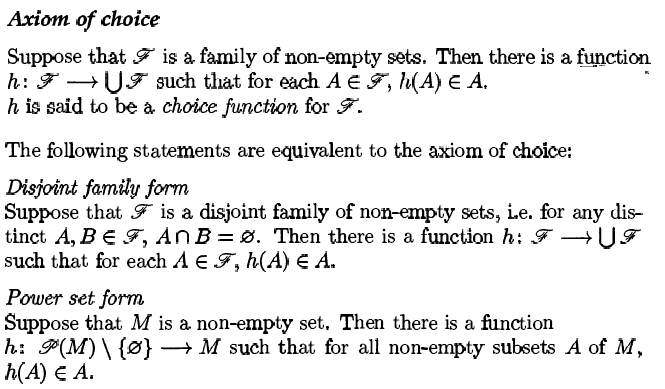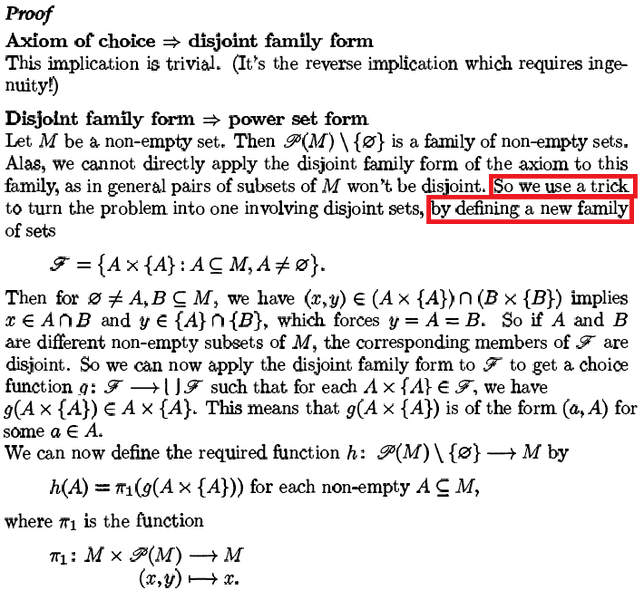# Axiom of Choice: Disjoint Family ##\Rightarrow ## Power Set

• I
• Terrell
In summary, the author of the proof used a trick to derive a power set form for a set which does not have a power set form. This trick is valid, as it is a defined family.f

#### Terrell

So apparently the proof involves a trick that converts the problem of a general power set ##\mathscr{P}(M)## of some set ##M## which has of course the property of not having pairwise disjoint set-elements to a problem that involves disjoint set-elements. I do not understand why this trick is valid because I think by doing so, we are then "re-proving" the case where the set-elements are disjoint.#### Attachments

Last edited:
It would have been helpful to know the precise wording of all three versions, for otherwise we can only guess.

Now, what if the author wouldn't have called it a trick and simply defined the family ##\mathcal{F}##? Would you still ask, if this is a valid family in the sense of the theorem?

It would have been helpful to know the precise wording of all three versions, for otherwise we can only guess.
let me put it up in a minute.

Would you still ask, if this is a valid family in the sense of the theorem?
I would think so since it is a defined family ##\mathscr{F}## then it would not be an arbitrary family..?

Last edited:
I don't see that an arbitrary family is needed. We need an arbitrary power set, which we have. Then we apply the disjoint version on ##\mathscr{F}##, for which we do not need arbitrariness. And finally we turn back on what it means for ##\mathscr{P}(M)##.

Then we apply the disjoint version on F
So the defined ##\mathscr{F}## is the disjoint version of ##\mathscr{P}(M)##?

As far as I can understand, not knowing the precise definitions of either of them. The structure is as follows:
• To be proven: power set form
• given: any (arbitrary) power set ##\mathscr{P}(M)##
• given disjoint family form is true for any disjoint family
• define ##\mathscr{F}##
• apply disjoint family form on ##\mathscr{F}##: as it is valid for all families of disjoint sets, it is also valid for ##\mathscr{F}##
• deduce power set form for ##\mathscr{P}(M)##

•Terrell
• define ##\mathscr{F}##
• apply disjoint family form on ##\mathscr{F}##: as it is valid for all families of disjoint sets, it is also valid for ##\mathscr{F}##
• deduce power set form for ##\mathscr{P}(M)##
So this "trick" is a set up so that we can formally deduce the power set form. Correct? I guess, I got so fixated in the "jump" in logic as to how the author of the proof have derived the "trick".

So this "trick" is a set up so that we can formally deduce the power set form. Correct?
Yes. The trick is, that we can only apply the disjoint family form, so we define ##\mathscr{F}## and make it applicable. We then still have to prove the power set form from that.

•Terrell
A bit unrelated. Do you think this trick could have been derived by working backwards? Anyway, thank you!

I'm not quite sure what you mean by backwards. It's more like "If you are not willing, then I need violence". One can ask: If I only may apply disjoint, but my power set isn't, how can I make it fit? Don't know, whether this can be called backwards, but it is what's going on.

•Terrell